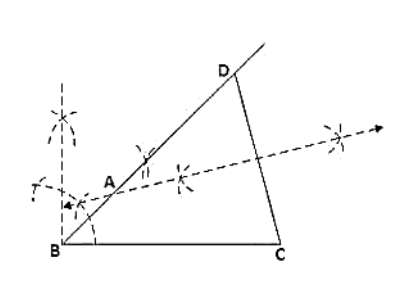# Construct a △ABC in which AB + AC = 5.6 cm, BC = 4.5 cm and ∠B = 45°.

Question:

Construct a △ABC in which AB + AC = 5.6 cm, BC = 4.5 cm and ∠B = 45°.

Solution:

Steps of Construction:

1. Construct a line segment BC of 4.5 cm.

2. At the point B, draw ∠XBC = 45°.

3. Keeping B as centre and radius 5.6cm draw an arc which intersects XB at D.

4. Join DC.

5. Draw the perpendicular bisector of DC which intersects DB at A.

6. Join AC.

Hence △ABC is the required triangle